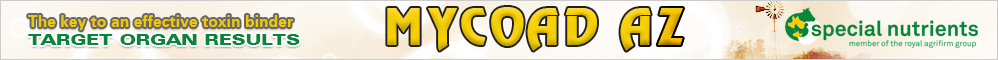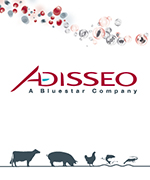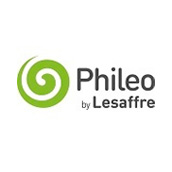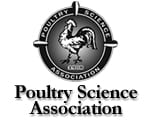# Use of nonlinear programming to determine the economically optimal energy density in laying hens diet during phase 2

Published on: 10/15/2020
Author/s :
Summary

A nonlinear programming Excel Workbook (Microsoft Corporation, Redmond, WA) was developed using the Excel Solver (Frontline Systems Inc., Incline Village, NV) to optimize energy density and bird performance. In this study, 6 dietary treatments (2.535, 2.635, 2.735, 2.835, 2.935, and 3.035 Mcal of ME/kg) were fed to Hy-Line W-36 laying hens (n = 192) in phase 2 (from 32 to 44 wk of age). Data were fitted to quadratic equations to express egg mass, feed consumption, and the objective function return over feed cost in terms of energy density. To demonstrate the capabilities of the model, the prices for eggs, corn, and soybean meal were increased and decreased by 25% and the program was solved for the maximum profit and optimized feed mix. By increasing the egg price, the model changed the optimal diet formulation and energy density in such a way as to improve performance and feed consumption, and accepted a higher energy concentration. Therefore, egg producers can realize considerable savings from using the nonlinear programming model described here, as opposed to a linear programming model with fixed minima for energy and other nutrients. These savings result from the ability of the nonlinear programming models to determine the most profitable energy density that should be fed as energy and protein prices change.

Key words: energy, feed formulation, laying hen, nonlinear programming, optimization.

DESCRIPTION OF PROBLEM

The main interests of animal nutritionists are in biology, not econometrics. As a result, the economic interpretation of feeding practices has practically been ignored in animal nutrition texts and in teaching programs. The development and implementation of easy, customized software models should facilitate the teaching of (and increase interest in) the economic ramifications of choices in animal nutrition and feeding .

The concept of feeding economically optimal concentrations of nutrients based on diminishing returns functions is not new but has rarely been used in nutrition . Linear programming is an effective method of finding the least possible cost of a unit of diet that satisfies a set of nutrient specifications. The commonly applied least-cost feed mix does not take productivity into account, nor does it use productivity information in setting energy and protein levels in response to their prices . Static methods of diet formulation ignore the importance of economics and are not adequate to optimize the economic returns on the process of egg production. Reducing feed costs may make the cost side of the equation look attractive, but the resulting loss in performance may have negative effects on profitability. Because the response of birds to energy density is a diminishing returns phenomenon, it should be evaluated economically to estimate an economic optimal level rather than a biological maximum. Roland et al.  demonstrated that nutrient requirements for maximum profits vary. Producers must let egg, protein, and energy prices dictate nutrient requirements. Because egg and feed prices change, there can be no fixed nutrient requirements.

Guevara  reported that nonlinear programming can be more useful than conventional linear programming in optimizing the performance response to energy density in broiler feed formulations because an energy level does not need to be set. At present, there is no reliable dynamic computer method of diet formulation to determine how changing prices of egg and feed ingredients affect performance and dietary energy density that maximizes margin over feed cost. Sterling et al.  used a quadratic programming model to determine economically optimal dietary CP and Lys levels for broiler chicks and demonstrated that maximum profit models would never result in less profit than conventional least-cost formulation models. The objective of our study was to evaluate the efficiency of a nonlinear programming optimization model to determine the effect of variation in ingredients and egg prices on the optimal energy density and feed mix and the margin over feed cost.

MATERIALS AND METHODS

In this experiment, Hy-Line W-36 hens (n = 192)   in phase 2 (32 to 44 wk of age) were randomly divided into 6 treatments (4 replicates of 8 birds per treatment). Replicates were equally distributed into upper and lower cages to minimize the cage level effect. This research project was carried out in compliance with the Guide to the Care and Use of Experimental Animals of the Canadian Council on Animal Care . All hens were supplied with feed and water ad libitum. Dietary treatments included the following energy densities: 2.535, 2.635, 2.735, 2.835, 2.935, and 3.035 Mcal of ME/kg. The 2.935 energy level was computer-formulated to meet the requirements of the Hy-Line Commercial Management Guide . Nutrient levels were kept at a constant ratio to energy level. Egg production and egg weight were recorded daily and feed consumption was recorded weekly. Egg mass and feed conversion (g of feed/g of egg) were calculated from egg production, egg weight, and feed consumption. Data were fitted to quadratic equations by using a Microsoft Excel  polynomial regression to express egg mass and feed consumption in terms of energy density. The optimization model and conventional linear programming were solved using the Solver, which is the default solver of Excel . It uses the generalized reduced gradient method to solve nonlinear problems. The options, which are specified by the user, were set as follows: iterations = 1,000, precision = 0.00001, convergence =  0.001, estimates = tangent, derivatives = forward, and search = Newton.

The feed formulation model used in this study has been detailed by Guevara  and is described here only briefly. This program takes the technically derived equation for egg mass, adds economic data on the cost of feed ingredients and the value of eggs, and calculates the density of energy that maximizes profit (return over feed cost). The quadratic production function and feed consumption were derived from the response of the birds to various dietary energy densities as

EM = a + bE − cE2, and

F = d − eE + fE2,

where EM is egg mass (kg), E is energy density (Mcal/kg), F is feed intake (kg), and a, b, c, d, e, and f are constants. These functions were used to create windows for economic modeling in a Microsoft Excel 2003 worksheet . The non-linear programming model is listed in Table 1.

The model identifies the combination of feed ingredients that maximize the return over feed cost. The linear programming matrix of Pesti et al.  was adapted to take energy density expressed as a ratio and bird response into account. Nutrient concentrations were kept at a constant ratio with energy level, and each nutrient constraint expressed as a ratio was transformed into equivalent linear constraints before using nonlinear programming. Energy density was entered as an extra ingredient in the left-hand side of the model. Because the nutrient:energy ratio is equal to the requirement divided by energy level, then the requirement on the right-hand side of the model is equivalent to the ratio times the energy level and could pass to the left-hand side of the model as a linear function. The effects of changes in different variables on the optimal energy density, diet formulation, performance, and profitability were performed using the non-linear programming model and were compared with conventional linear programming. To demonstrate the capabilities of the model, the prices for eggs, corn, and soybean meal were increased and decreased by 25%, and the program was solved for the maximum profit and optimized feed mix. Formulations were identical in all other respects. The nonlinear programming matrix and constraint set used in optimization are shown in Table 2.

RESULTS AND DISCUSSION

Egg mass and feed consumption response functions are shown in Figures 1 and 2, respectively. The objective function obtained was

margin = egg price × [(0.25 × E2)

−  (0.5754 × E) + 4.1427]

– {feed cost×[(−0.6786 × E2)

+  (1.2368 × E) + 10.542]},

where E is energy density (Mcal/kg).

The effect of changing prices of eggs, corn, and soybean meal on the optimal performance and energy density are shown in Table 3. The profit margin was much higher when the prices of ingredients were low. By increasing the egg price, the model changed the optimal diet formulation and energy density in such a way as to improve performance and feed consumption and accepted a higher energy concentration. As the price of soybean meal increased, its dietary usage decreased, and the model increased the optimal energy density and optimized the diet composition that leads to improved feed conversion and bird performance. As the price of soybean meal increased, alternate protein sources could be drawn in. Presumably, the costs of alternate protein sources would increase at the same time as soybean meal, so that might limit the substitutions and savings observed.

In Table 4, the least-cost feed formulation (linear programming method) is compared with the maximum profit formulation using the non-linear programming model. The margin and diet cost changed compared with those calculated by conventional linear programming, with the diet formulated using nonlinear programming being more profitable. If the maximum profit formulation model is used, the economically optimal energy density may change depending on the prices of energy and the value of eggs.

Our results follow the law of diminishing returns and basic principles of production economics. If the price of the inputs increases, their use tends to decrease; if the value of the product increases, the level of output tends to increase, and the converse. Based on these results, egg producers appear to be able to have considerable savings from using the nonlinear programming model described here, as opposed to a linear programming model with fixed minima for energy and other nutrients. These savings result from the ability of nonlinear programming models to determine the most profitable energy density that should be fed as energy and protein prices change. Linear programming models contain no features that allow them to make this determination . The nonlinear model workbook gives producers a working tool to demonstrate the interdependencies of costs and technical response functions and the value of eggs.

The nonlinear model may be used not only for optimization, but also for evaluation of the current feeding situation. In this way, bird performance estimated by the model can be compared with the actual performance observed on the farm to evaluate the efficiency of current feeding practices.

Wu et al.  demonstrated that the strain of hen has a significant effect on egg mass. It is important that producers develop their production functions with the genetic stocks and under the conditions they will be using commercially.

CONCLUSIONS AND APPLICATIONS

1. The nonlinear model workbook gives producers a working tool to demonstrate the interdependencies of costs and technical response functions and the value of eggs.

2. Egg producers are able to have considerable savings from using the nonlinear programming model in diet formulations, as opposed to a linear programming model with fixed minima for energy and other nutrients.

3. The nonlinear model may be used not only for optimization, but also for evaluation of the current feeding situation as well.

4. Producers must develop their production functions with the genetic stocks and under the conditions they will be using commercially.

Bibliographic references

Author/s

remove_red_eye 32 forum 0 bar_chart Statistics share print
Share :
close|